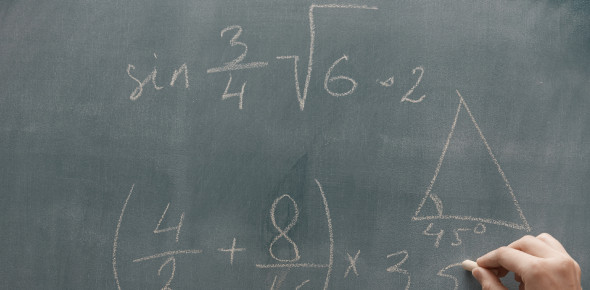# Do You Know Dividing Decimals? Quiz

10 Questions | Attempts: 301
ShareSettingsHow good are you with numbers? Do you know diving decimals? Why don't you take this quiz, and we will get to see how many questions you are able to answer about dividing decimals? The decimal numeral system is known as the standard system for denoting integer and non-integer numbers. This is what most all math lovers know. However, this quiz will enlighten you even more about dividing decimals. All the best to you.

• 1.
Dividing Decimals is a topic under ...?
• A.

Mathematics

• B.

Music

• C.

Biology

• D.

Literature

• 2.
Decimals are usually taught at ...?
• A.

Church

• B.

Mosque

• C.

Museum

• D.

School

• 3.
If the divisor is not a whole number, move the decimal point to .... to make it a whole number.
• A.

Right

• B.

Left

• C.

Top

• D.

Middle

• 4.
Dividing Decimals has to do with ...
• A.

Multiplication

• B.

• C.

Subtraction

• D.

Division

• 5.
To divide decimal numbers, put decimal point directly ... decimal point in the dividend.
• A.

Above

• B.

Below

• C.

Middle

• D.

None

• 6.
The number we divide by is called ... ?
• A.

Divider

• B.

Divisor

• C.

Calculator

• D.

Equator

• 7.
To divide decimal numbers, multiply the divisor by as many ...
• A.

10's

• B.

20's

• C.

5's

• D.

30's

• 8.
Dividing Decimals is a sub-topic under ...?
• A.

Fractions

• B.

Decimals

• C.

Algebra

• D.

Ratio

• 9.
Decimals are _____ in math.
• A.

Useful

• B.

Useless

• C.

Myth

• D.

Rare

• 10.
Which of these is not a decimal number?
• A.

1782.1

• B.

0.182

• C.

27.2

• D.

567

## Related Topics

×

Wait!
Here's an interesting quiz for you.